Note

This page is a reference documentation. It only explains the class signature, and not how to use it. Please refer to the user guide for the big picture.

# nilearn.connectome.ConnectivityMeasure#

A class that computes different kinds of functional connectivity matrices.

New in version 0.2.

Parameters:
cov_estimatorestimator object, optional.

The covariance estimator. By default the LedoitWolf estimator is used. This implies that correlations are slightly shrunk towards zero compared to a maximum-likelihood estimate

kind{“covariance”, “correlation”, “partial correlation”, “tangent”, “precision”}, optional

The matrix kind. For the use of “tangent” see . Default=’covariance’.

vectorizebool, optional

If True, connectivity matrices are reshaped into 1D arrays and only their flattened lower triangular parts are returned. Default=False.

If True, vectorized connectivity coefficients do not include the matrices diagonal elements. Used only when vectorize is set to True. Default=False.

References

Attributes:
`cov_estimator_`estimator object

A new covariance estimator with the same parameters as cov_estimator.

`mean_`numpy.ndarray

The mean connectivity matrix across subjects. For ‘tangent’ kind, it is the geometric mean of covariances (a group covariance matrix that captures information from both correlation and partial correlation matrices). For other values for “kind”, it is the mean of the corresponding matrices

`whitening_`numpy.ndarray

The inverted square-rooted geometric mean of the covariance matrices.

fit(X, y=None)[source]#

Fit the covariance estimator to the given time series for each subject.

Parameters:
Xlist of numpy.ndarray, shape for each (n_samples, n_features)

The input subjects time series. The number of samples may differ from one subject to another.

Returns:
selfConnectivityMatrix instance

The object itself. Useful for chaining operations.

fit_transform(X, y=None, confounds=None)[source]#

Fit the covariance estimator to the given time series for each subject. Then apply transform to covariance matrices for the chosen kind.

Parameters:
Xlist of n_subjects numpy.ndarray with shapes (n_samples, n_features)

The input subjects time series. The number of samples may differ from one subject to another.

confoundsnp.ndarray with shape (n_samples) or (n_samples, n_confounds), or pandas DataFrame, optional

Confounds to be cleaned on the vectorized matrices. Only takes into effect when vetorize=True. This parameter is passed to signal.clean. Please see the related documentation for details.

Returns:
outputnumpy.ndarray, shape (n_subjects, n_features, n_features) or (n_subjects, n_features * (n_features + 1) / 2) if vectorize is set to True.

The transformed individual connectivities, as matrices or vectors. Vectors are cleaned when vectorize=True and confounds are provided.

transform(X, confounds=None)[source]#

Apply transform to covariances matrices to get the connectivity matrices for the chosen kind.

Parameters:
Xlist of n_subjects numpy.ndarray with shapes (n_samples, n_features)

The input subjects time series. The number of samples may differ from one subject to another.

confoundsnumpy.ndarray with shape (n_samples) or (n_samples, n_confounds), optional

Confounds to be cleaned on the vectorized matrices. Only takes into effect when vetorize=True. This parameter is passed to signal.clean. Please see the related documentation for details.

Returns:
outputnumpy.ndarray, shape (n_subjects, n_features, n_features) or (n_subjects, n_features * (n_features + 1) / 2) if vectorize is set to True.

The transformed individual connectivities, as matrices or vectors. Vectors are cleaned when vectorize=True and confounds are provided.

inverse_transform(connectivities, diagonal=None)[source]#

Return connectivity matrices from connectivities, vectorized or not.

If kind is ‘tangent’, the covariance matrices are reconstructed.

Parameters:
connectivitieslist of n_subjects numpy.ndarray with shapes (n_features, n_features) or (n_features * (n_features + 1) / 2,)

or ((n_features - 1) * n_features / 2,) Connectivities of each subject, vectorized or not.

diagonalnumpy.ndarray, shape (n_subjects, n_features), optional

The diagonals of the connectivity matrices.

Returns:
outputnumpy.ndarray, shape (n_subjects, n_features, n_features)

The corresponding connectivity matrices. If kind is ‘correlation’/ ‘partial correlation’, the correlation/partial correlation matrices are returned. If kind is ‘tangent’, the covariance matrices are reconstructed.

get_params(deep=True)#

Get parameters for this estimator.

Parameters:
deepbool, default=True

If True, will return the parameters for this estimator and contained subobjects that are estimators.

Returns:
paramsdict

Parameter names mapped to their values.

set_output(*, transform=None)#

Set output container.

See Introducing the set_output API for an example on how to use the API.

Parameters:
transform{“default”, “pandas”}, default=None

Configure output of transform and fit_transform.

• “default”: Default output format of a transformer

• “pandas”: DataFrame output

• None: Transform configuration is unchanged

Returns:
selfestimator instance

Estimator instance.

set_params(**params)#

Set the parameters of this estimator.

The method works on simple estimators as well as on nested objects (such as `Pipeline`). The latter have parameters of the form `<component>__<parameter>` so that it’s possible to update each component of a nested object.

Parameters:
**paramsdict

Estimator parameters.

Returns:
selfestimator instance

Estimator instance.

## Examples using `nilearn.connectome.ConnectivityMeasure`#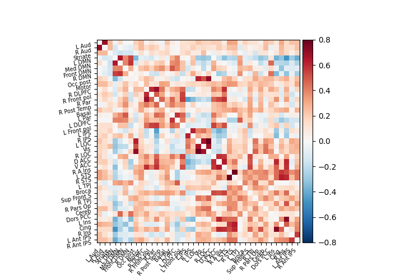Extracting signals of a probabilistic atlas of functional regions

Extracting signals of a probabilistic atlas of functional regions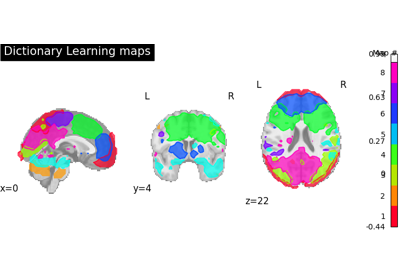Regions extraction using dictionary learning and functional connectomes

Regions extraction using dictionary learning and functional connectomes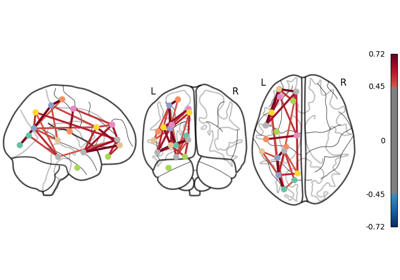Comparing connectomes on different reference atlases

Comparing connectomes on different reference atlases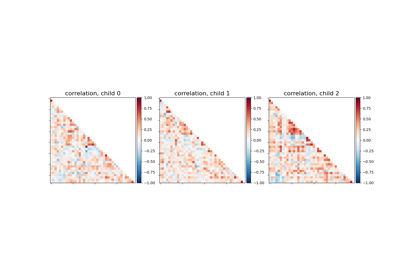Classification of age groups using functional connectivity

Classification of age groups using functional connectivity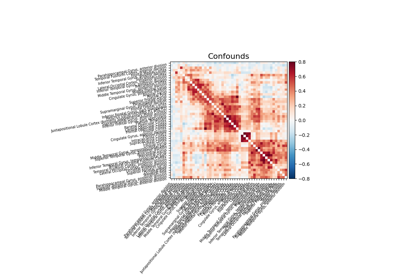Extracting signals from a brain parcellation

Extracting signals from a brain parcellation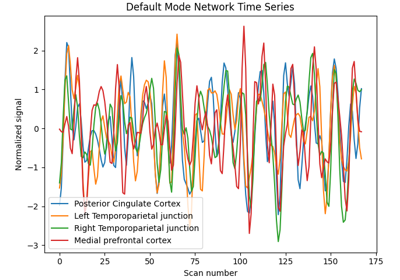Extract signals on spheres and plot a connectome

Extract signals on spheres and plot a connectome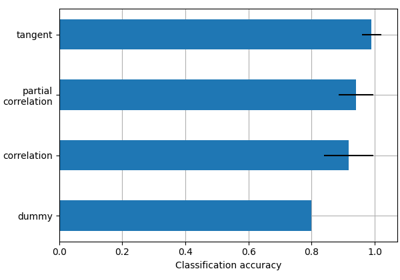Functional connectivity predicts age group

Functional connectivity predicts age group